## how to convert sq ft to yard

Don't know how to calculate the area of your garden or circumference of a pond? Ask your question here.
Forum rules
Dear convert-me.com forum visitors,

Our forum has been available for many years. In September 2014 we decided to switch it to read-only mode. Month after month we saw less posts with questions and answers from real people and more spam posts. We were spending more and more resources cleaning the spam until there were less them 1 legitimate message per 100 spam posts. Then we decided it's time to stop.

All the posts in the forum will be available and searchable. We understand there are a lot of useful information and we aren't going to remove anything. As for the new questions, you can always ask them on convert-me.com FaceBook page

Thank you for being with us and sorry for any inconveniences this could caused.

### how to convert sq ft to yard

hi.

i want to convert sq feet to sq yard. How do i do it?
how many feet form a yard???

i want to buy a carpet and my room is 13 by 10 sq ft. but i am getting carpet in yards
so how do i know how much i would need
guest123

### Re: how to convert sq ft to yard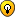Answers to your questions are here: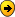How may feet are in a yard?How may square feet are in a square yard?

----------------------------------------
guest123 wrote:hi.

i want to convert sq feet to sq yard. How do i do it?
how many feet form a yard???

i want to buy a carpet and my room is 13 by 10 sq ft. but i am getting carpet in yards so how do i know how much i would need

For converting measurements of area (e.g. square feet to square yeards), use this page: http://www.convert-me.com/en/convert/area

One square yard = 9 square feet.
One yard (linear yard) = 3 feet (linear feet)

If your room is 13 by 10 feet (not square feet!), its area is 13*9=113 square feet = 12.56 square yards.
Last edited by convert-me.com on Sat Jan 21, 2006 12:32 pm, edited 1 time in total.
Convert-Me.Com - Interactive Units Conversion
http://www.convert-me.com/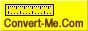convert-me.com
Site Admin

Posts: 402
Joined: Fri Sep 05, 2003 4:50 pm

While the provided formula is technically correct, please note that most carpet centers are not willing to cut a piece of carpet for you that will measure your exact measurements of 13' x 10'. Instead, you are probably buying a carpet that is 12' wide x 13' long. Carpets tend to be either 6' or 12' across, and since they will have such a difficult time selling your remnant carpet measuring 2' x 13', they will "give" you that portion along with the carpet you are actually purchasing. Your actual purchase price will be based of the 12' wide x 13' long or 17.33 square yards (12' x 13' = 156 square feet / 9 square feet/yard = 17.33 square yards).
Conquest

Posts: 12
Joined: Wed Dec 08, 2004 12:34 am
Location: Raymond MS - USA

### Re: how to convert sq ft to yard

[
If your room is 13 by 10 feet (not square feet!), its area is 13*9=113 square feet = 12.56 square yards.[/quote]
Guest

### yards

hi, i would like to know. How many feet are in ayard ?
guest1981

### fert and yards

How many feet are in a yard??[/b]
Cokiemonsterbabe

### Re: yards

guest1981 wrote:hi, i would like to know. How many feet are in ayard ?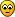there are 9 square feet in a square yard
Guest

### Re: yards

Anonymous wrote:
guest1981 wrote:hi, i would like to know. How many feet are in ayard ?there are 9 square feet in a square yard

3 feet in a lin. yardGuest

### MATH

hOW MANY SQUARE FEET ARE IN A SQUARE YARD?
mamasguy

### buying carpet

my room is 12 x 15 how many yards of carpet will i need please help..
Guest

### Re: how to convert sq ft to yard

24 feet equals how many yards
Guest

### sq. feet in a sq. yard

anyone who doesn't know how many square feet there are in a square yard shouldn't be buying carpet *l*
wils

can any1 guess how long it would take 2 travel, 157km in hours in a car?
Guest

13x10 room
carpet sold 12ft wide
purchase 12x13=156sq.ft
156sq ft divided by 9 = 17.33 sq yards

You have to trim the 2'' off the 12"
mdm

### Re: How do I convert sq yards to lineal yards

how many yards is in a foot
may

I would like to buy decking - the measurement for the area is 11 ft x 14ft and the area of each decking is 17 3/4 x 17 3/4". Please could you work out how many decking I would need to buy - thanks you
domadoma

### question

guys could you please answer this for me. How do I convert my room measurement which is 13 by 13 to sq feet?
newbie

### carpeting room

how many square yards are in a room measuring 15' by 18' feet
ables

To convert your 13 x 13 room is somewhat easy. Carpet only comes in 12ft rolls. So you first start with a 12ft x 13ft piece of carpet. Now you have a 1 foot by 13 foot piece that needs to be filled. Depending on how many seams you want will determine the amount of carpet you will need. Normally this is done by using 3 foot lengths. So now you room's size is 12ft x 16ft to give you enough carpet to cover the full 13 x 13. Now let translate this into square yards. If you take the overall length and make it to inches 16ft =192 inches. Take the 192 and divide it by 9 inches(the amount of carpet to equal 1 yard at 12 ft roll length) which will give you 21.33 , so this means your room will require 21 1/3 yards to cover the floor with 5 total seams. The more carpet you buy the less seams you will have, but the more it will cost.
Guest

### Re: carpeting room

ables wrote:how many square yards are in a room measuring 15' by 18' feet

To do this will require a little more. Remember that carpet normally comes in 12 foot rolls, but some can come in 13.5 and 15 ft rolls. But here goes. Depending on what you buy will determine the true square yards. If your carpet has no pattern to match here is your answer 32 yards with 3 total seams.
Guest

### Re: how to convert sq ft to yard

guest123 wrote:hi.

i want to convert sq feet to sq yard. How do i do it?
how many feet form a yard???

i want to buy a carpet and my room is 13 by 10 sq ft. but i am getting carpet in yards
so how do i know how much i would need

Here is your answer to this one. Remember that carpet usually comes in 12 ft rolls so you will end up with some let over so your room is actually in carpet is a 12 x 13. Now take the 13 and divide it by 3 and add that amount to the 13 and you get the total sqaure yardage you will need.
13/3= 4.33 + 13 = 17.33 yards.

Hope this helped.
Guest

### Re: buying carpet

Anonymous wrote:my room is 12 x 15 how many yards of carpet will i need please help..

12 x 15 equates to 15/3=5+15= 20 sq yrds
Guest

### Re: perimeter and square feet

help me to understand why 40*40 square feet = 1600 and the perimetrer or the linear feet is 40+40+40+40 = 160 and as you continue to use the same perimeter such as 30*50 or 20*60 or any combination that amounts to 80 all the perimeter is always 160 but the square feet changes but why doesn't the perimeter or the linear doesn't change ?
myers

### Re: perimeter and square feet

myers wrote:help me to understand why 40*40 square feet = 1600 and the perimetrer or the linear feet is 40+40+40+40 = 160 and as you continue to use the same perimeter such as 30*50 or 20*60 or any combination that amounts to 80 all the perimeter is always 160 but the square feet changes but why doesn't the perimeter or the linear doesn't change ?

To understand it try a simple game. Take a lace and tie its ends together. That's your perimeter. Now put it on the table so it makes a circle. Note the area inside. Next line it up so that it makes a long narrow bar. Note again the area inside the lace. It became much smaller now while perimeter remained the same.

The experiment above illustrates the fact that area and perimeter are very loosely related.
Convert-Me.Com - Interactive Units Conversion
http://www.convert-me.com/convert-me.com
Site Admin

Posts: 402
Joined: Fri Sep 05, 2003 4:50 pm

### Lineal yard to square feet

How do you convert a number in lineal yards to square feet?
Guest

### Re: Lineal yard to square feet

Guest wrote:How do you convert a number in lineal yards to square feet?

Read this: http://sergey.gershtein.net/blog/2006/1 ... -foot.html
Convert-Me.Com - Interactive Units Conversion
http://www.convert-me.com/convert-me.com
Site Admin

Posts: 402
Joined: Fri Sep 05, 2003 4:50 pm

### Re: buying carpet

Anonymous wrote:
Anonymous wrote:my room is 12 x 15 how many yards of carpet will i need please help..

12 x 15 equates to 15/3=5+15= 20 sq yrds

Or you can figure it this way.

12 x 15= 180sq ft. To figure sq yrds by sq ft, divide by 9= 20sq yrdsGuest

It seems like kids are asking answers to their math problems!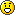Guest

### Re: how to convert sq ft to yard

guest123 wrote:hi.

i want to convert sq feet to sq yard. How do i do it?
how many feet form a yard???

i want to buy a carpet and my room is 13 by 10 sq ft. but i am getting carpet in yards
so how do i know how much i would need

= 130 sq ft and convert to yds is = 14.44 sq yds.
There are 9 sq ft in 1 sq yd so multiply room width by lenght for sq ft and the divide that by 9 to get your yards.
shelby4632

### Re: buying carpet

Anonymous wrote:my room is 12 x 15 how many yards of carpet will i need please help..
12X15=180=20
Guest

Hi my daughter's room is 10 foot long by 8 foot wide
how many s.q.y.d would i need in carpet for it
hope somebody can help me Thank you
Kindest Regards TinaGuest

Anonymous wrote:Hi my daughter's room is 10 foot long by 8 foot wide
how many s.q.y.d would i need in carpet for it
hope somebody can help me Thank you
Kindest Regards Tina10' x 8' = 80 ft^2
80 ft^2 / 9 ft^2 = 8.89 yd^2, so 9 square yards of carpet.

Now the problem (you knew there'd be a problem). Most carpet is sold in 13 feet widths so don't just go out and get 9 yards of carpet as it'll be too short in one dimension and too long in the other.

You might be able to find a remnant at a carpet store that will work but wherever you buy the carpet, give the tech your dimensions in feet to be sure you get enough carpet.

I strongly suggest you have the carpet professionally installed.
Dirtman
Expert

Posts: 574
Joined: Sat Jan 21, 2006 2:04 pm
Location: California

### linear yard

Hi. I need help, I dont understand linear yard. How long is it? in meters or feet.
Hoping for your kind response.
Thank you.
vertigoth

### Re: linear yard

vertigoth wrote:Hi. I need help, I dont understand linear yard. How long is it? in meters or feet.
Hoping for your kind response.
Thank you.

The word "linear" is often over used. When dealing with length, the word "linear" usually doesn't need to be stated.

Linear (adj.):
Of, relating to, or resembling a line; straight or curved.
1. In, of, along, describing, described by, or related to a straight or curved line: a â€ślinear footâ€ť.
2. Having only one dimension.

So, a linear yard is simply a line that's 1 yard long (36"). A linear foot is a line that's 1 foot long (12").

Does that help?
Dirtman
Expert

Posts: 574
Joined: Sat Jan 21, 2006 2:04 pm
Location: California

### reply about "linear"

Thank you DIRTMAN.

That says it all. I fully understand it now.
vertigoth

### Re: perimeter and square feet

convert-me.com wrote:
myers wrote:help me to understand why 40*40 square feet = 1600 and the perimetrer or the linear feet is 40+40+40+40 = 160 and as you continue to use the same perimeter such as 30*50 or 20*60 or any combination that amounts to 80 all the perimeter is always 160 but the square feet changes but why doesn't the perimeter or the linear doesn't change ?

To understand it try a simple game. Take a lace and tie its ends together. That's your perimeter. Now put it on the table so it makes a circle. Note the area inside. Next line it up so that it makes a long narrow bar. Note again the area inside the lace. It became much smaller now while perimeter remained the same.

The experiment above illustrates the fact that area and perimeter are very loosely related.

WRONG....If you tie a lace together and make a circle, it will have the EXACT inside area as if you were to make a square, triangle, or narrow bar.
Guest

### Re: perimeter and square feet

Anonymous wrote:
convert-me.com wrote:
myers wrote:help me to understand why 40*40 square feet = 1600 and the perimetrer or the linear feet is 40+40+40+40 = 160 and as you continue to use the same perimeter such as 30*50 or 20*60 or any combination that amounts to 80 all the perimeter is always 160 but the square feet changes but why doesn't the perimeter or the linear doesn't change ?

To understand it try a simple game. Take a lace and tie its ends together. That's your perimeter. Now put it on the table so it makes a circle. Note the area inside. Next line it up so that it makes a long narrow bar. Note again the area inside the lace. It became much smaller now while perimeter remained the same.

The experiment above illustrates the fact that area and perimeter are very loosely related.

WRONG....If you tie a lace together and make a circle, it will have the EXACT inside area as if you were to make a square, triangle, or narrow bar.

Wrong? Sorry, but Sergey (Convert-me.com) is correct.

Examples with a perimeter of 200 feet:
Square = 2500 ft2
Circle = 3183.1 ft2
Equilateral Triangle = 1924.5 ft2
90' * 10' Narrow Rectangle = 900 ft2

And all have a 200' perimeter!
Dirtman
Expert

Posts: 574
Joined: Sat Jan 21, 2006 2:04 pm
Location: California

### Re: how to convert sq ft to yard

Hi..
Thanks for sharing the post and its really good information..
stevejack

Posts: 1
Joined: Thu Oct 08, 2009 10:10 am

### Re: how to convert sq ft to yard

I have a room which is 12ft by 15ft and need to know what size carpet in metres i need. Could someone please help me? Thank you
wendi

### Re: how to convert sq ft to yard

1 foot = 0.3048 meters
So, it's nearly 3,65m x 5 m if you want to cover the whole floorLast edited by Liandella on Fri Jun 15, 2012 1:08 pm, edited 2 times in total.
Liandella

Posts: 1
Joined: Tue Sep 27, 2011 5:09 pm

### Re: how to convert sq ft to yard

There are many free tools on the web that help with this.
rugmen

### Re: how to convert sq ft to yard

I have a Carpet cleaning company and when giving out prices over the phone for carpet cleaning I always have a problem converting sq ft to yards , what is the formula
Last edited by Dmreed4311 on Sat Nov 05, 2011 7:13 pm, edited 1 time in total.
Dmreed4311

Posts: 2
Joined: Mon Oct 24, 2011 3:06 am

### Re: how to convert sq ft to yard

ok here are two little tables that should solve the problem

sq ft = sq yd

1.0 = 0.11111
2.0 = 0.22222
3.0 = 0.33333
4.0 = 0.44444
5.0 = 0.55556
6.0 = 0.66667
7.0 = 0.77778
8.0 = 0.88889
9.0 = 1.00000

sq yd = sq ft

1.0 = 9.00000
2.0 = 18.00000
3.0 = 27.00000
4.0 = 36.00000
5.0 = 45.00000
6.0 = 54.00000
7.0 = 63.00000
8.0 = 72.00000
9.0 = 81.00000

hope that helps

Sarah
Sarah1984

Posts: 1
Joined: Mon Oct 24, 2011 11:46 pm

### More info

• List of all units you can convert online
• Metric conversion
• Convert pounds to gallons
• Convert grams to cups
• Grams to milliliters
• Imperial vs US Customary
• History of measurement
• Return to Calculating things

 Our Privacy Policy Cooking Measures Converter Metric conversions